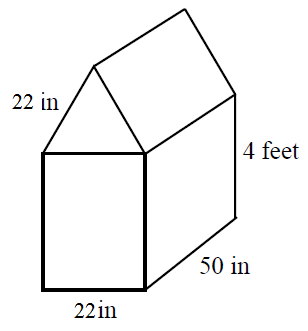# Surface area and volume of prisms

### Surface area and volume of prisms

So, we've learned the basics in the "Introduction to volume" lesson. Now, let's practice some harder questions on how to find out the surface area and volume of prisms and composite solids.

#### Lessons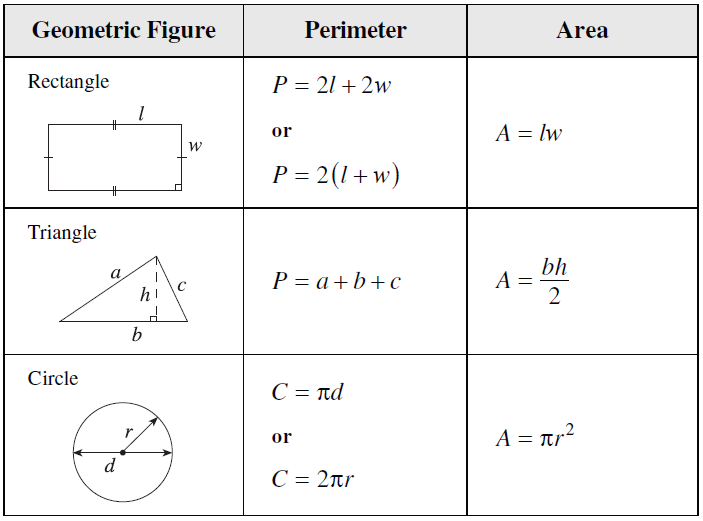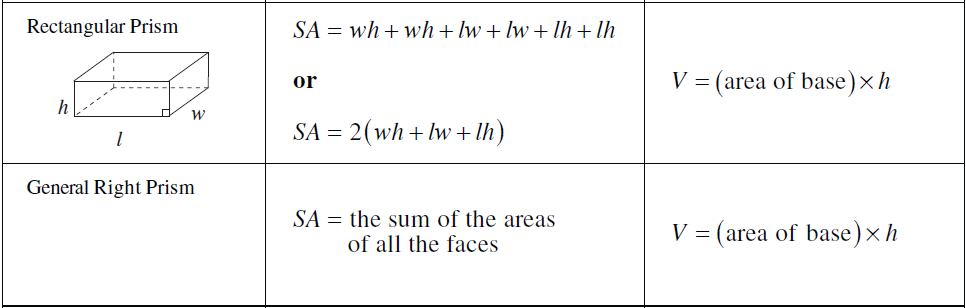• Introduction
a)
Intro to Volume
• What are prisms?
• What are cubes?

• 1.
Find the surface area and the volume of the following prisms:
a)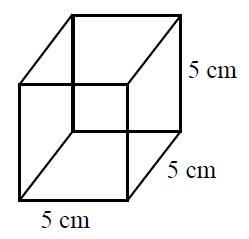b)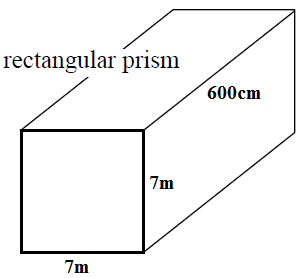c)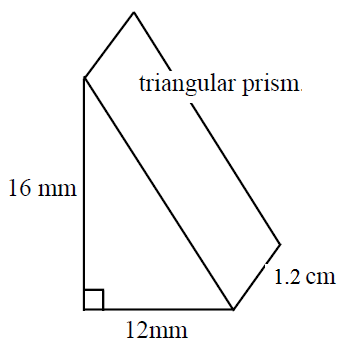d)